# [Explainer] Introduction and General Approach Final Pack!

Introduction to this challenge, general approach, my approach, and what I learn from the others

This is my final explainer trying to summarize what I know and learned from this challenge especially from other wonderful participants.

Notebook list:

I'll try to add more notebooks in the comment about my round 1 approach and what new things I learned (post-processing method, etc).

I hope you guys enjoy it and learn something from this explainer.

---

Thanks to SEAM AI and Aicrowd for organizing this event.

Also, shout out to other contestant's explainer :

This has been a wonderful experience, I'm glad that I spent my time for this challenge. Always checked the forum and leaderboard every time I got back from work.

I learned a lot from this community and was surprised that I can push my score to F1:0.901 Acc:0.941 (Round 1 Unweighted) and F1:0.770 Acc:0.737 (Round 2 weighted). I'm pretty noob at this.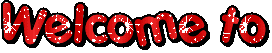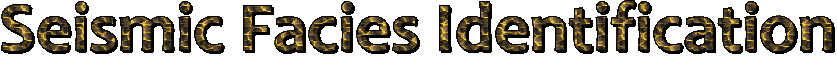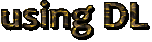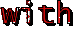# Shout-out¶

First of all, thank you for SEAM AI and Aicrowd for organizing this event.

Also shout out to other contestants explainer (I suggest you read it too) :

I learned a lot from this community and suprised that I can push my score to F1:0.901 Acc:0.941 (Round 1 Unweighted) and F1:0.770 Acc:0.737 (Round 2 weighted).

Also checkout my previous explainer too here :P

ps: for geoscientist, what I meant for processing here is to transform input data provided from this challenge.

# Introduction¶

In [ ]:
```#@title
from IPython.display import HTML,clear_output
from base64 import b64encode
clear_output()
data_url = "data:video/mp4;base64," + b64encode(mp4).decode()
HTML("""
<video width=480 controls>
<source src="%s" type="video/mp4">
</video>
""" % data_url)
```
`Output hidden; open in https://colab.research.google.com to view.`

Sorry for the tone difference lol, I only got time to do this while my family is asleep.

In [ ]:
```!gdown "https://drive.google.com/uc?id=14u7fkARS8WRJUdhvU79kDxg8EKTqg606"
```
```Downloading...
To: /content/data_train.npz
1.72GB [00:18, 94.5MB/s]
To: /content/labels_train.npz
7.16MB [00:00, 63.3MB/s]
```

Let's install some package that'll make our job easier :

In [ ]:
```!pip install segmentation-models-pytorch==0.1.2  # easy to use some famous model architecture. visit https://github.com/qubvel/segmentation_models.pytorch/
!pip install albumentations               # easy image manipulation for data augmentation
```
```Collecting segmentation-models-pytorch==0.1.2
|████████████████████████████████| 61kB 8.1MB/s
Requirement already satisfied: torchvision>=0.3.0 in /usr/local/lib/python3.6/dist-packages (from segmentation-models-pytorch==0.1.2) (0.8.1+cu101)
Collecting pretrainedmodels==0.7.4
|████████████████████████████████| 61kB 8.9MB/s
Collecting timm==0.1.20
|████████████████████████████████| 163kB 36.5MB/s
Collecting efficientnet-pytorch==0.6.3
Requirement already satisfied: torch==1.7.0 in /usr/local/lib/python3.6/dist-packages (from torchvision>=0.3.0->segmentation-models-pytorch==0.1.2) (1.7.0+cu101)
Requirement already satisfied: numpy in /usr/local/lib/python3.6/dist-packages (from torchvision>=0.3.0->segmentation-models-pytorch==0.1.2) (1.19.5)
Requirement already satisfied: pillow>=4.1.1 in /usr/local/lib/python3.6/dist-packages (from torchvision>=0.3.0->segmentation-models-pytorch==0.1.2) (7.0.0)
Collecting munch
Requirement already satisfied: tqdm in /usr/local/lib/python3.6/dist-packages (from pretrainedmodels==0.7.4->segmentation-models-pytorch==0.1.2) (4.41.1)
Requirement already satisfied: dataclasses in /usr/local/lib/python3.6/dist-packages (from torch==1.7.0->torchvision>=0.3.0->segmentation-models-pytorch==0.1.2) (0.8)
Requirement already satisfied: future in /usr/local/lib/python3.6/dist-packages (from torch==1.7.0->torchvision>=0.3.0->segmentation-models-pytorch==0.1.2) (0.16.0)
Requirement already satisfied: typing-extensions in /usr/local/lib/python3.6/dist-packages (from torch==1.7.0->torchvision>=0.3.0->segmentation-models-pytorch==0.1.2) (3.7.4.3)
Requirement already satisfied: six in /usr/local/lib/python3.6/dist-packages (from munch->pretrainedmodels==0.7.4->segmentation-models-pytorch==0.1.2) (1.15.0)
Building wheels for collected packages: pretrainedmodels, efficientnet-pytorch
Building wheel for pretrainedmodels (setup.py) ... done
Created wheel for pretrainedmodels: filename=pretrainedmodels-0.7.4-cp36-none-any.whl size=60963 sha256=15ecf5d77b10b7173e744ea8e29e484fd320dd124df60adf1fb7da5e67054433
Stored in directory: /root/.cache/pip/wheels/69/df/63/62583c096289713f22db605aa2334de5b591d59861a02c2ecd
Building wheel for efficientnet-pytorch (setup.py) ... done
Created wheel for efficientnet-pytorch: filename=efficientnet_pytorch-0.6.3-cp36-none-any.whl size=12421 sha256=d62b69be61ee765ae545f905231aa4a6576329cedad28cb4234bf47486d6586b
Successfully built pretrainedmodels efficientnet-pytorch
Installing collected packages: munch, pretrainedmodels, timm, efficientnet-pytorch, segmentation-models-pytorch
Successfully installed efficientnet-pytorch-0.6.3 munch-2.5.0 pretrainedmodels-0.7.4 segmentation-models-pytorch-0.1.2 timm-0.1.20
Requirement already satisfied: albumentations in /usr/local/lib/python3.6/dist-packages (0.1.12)
Requirement already satisfied: numpy>=1.11.1 in /usr/local/lib/python3.6/dist-packages (from albumentations) (1.19.5)
Collecting imgaug<0.2.7,>=0.2.5
|████████████████████████████████| 634kB 16.2MB/s
Requirement already satisfied: opencv-python in /usr/local/lib/python3.6/dist-packages (from albumentations) (4.1.2.30)
Requirement already satisfied: scipy in /usr/local/lib/python3.6/dist-packages (from albumentations) (1.4.1)
Requirement already satisfied: scikit-image>=0.11.0 in /usr/local/lib/python3.6/dist-packages (from imgaug<0.2.7,>=0.2.5->albumentations) (0.16.2)
Requirement already satisfied: six in /usr/local/lib/python3.6/dist-packages (from imgaug<0.2.7,>=0.2.5->albumentations) (1.15.0)
Requirement already satisfied: matplotlib!=3.0.0,>=2.0.0 in /usr/local/lib/python3.6/dist-packages (from scikit-image>=0.11.0->imgaug<0.2.7,>=0.2.5->albumentations) (3.2.2)
Requirement already satisfied: PyWavelets>=0.4.0 in /usr/local/lib/python3.6/dist-packages (from scikit-image>=0.11.0->imgaug<0.2.7,>=0.2.5->albumentations) (1.1.1)
Requirement already satisfied: networkx>=2.0 in /usr/local/lib/python3.6/dist-packages (from scikit-image>=0.11.0->imgaug<0.2.7,>=0.2.5->albumentations) (2.5)
Requirement already satisfied: pillow>=4.3.0 in /usr/local/lib/python3.6/dist-packages (from scikit-image>=0.11.0->imgaug<0.2.7,>=0.2.5->albumentations) (7.0.0)
Requirement already satisfied: imageio>=2.3.0 in /usr/local/lib/python3.6/dist-packages (from scikit-image>=0.11.0->imgaug<0.2.7,>=0.2.5->albumentations) (2.4.1)
Requirement already satisfied: cycler>=0.10 in /usr/local/lib/python3.6/dist-packages (from matplotlib!=3.0.0,>=2.0.0->scikit-image>=0.11.0->imgaug<0.2.7,>=0.2.5->albumentations) (0.10.0)
Requirement already satisfied: python-dateutil>=2.1 in /usr/local/lib/python3.6/dist-packages (from matplotlib!=3.0.0,>=2.0.0->scikit-image>=0.11.0->imgaug<0.2.7,>=0.2.5->albumentations) (2.8.1)
Requirement already satisfied: pyparsing!=2.0.4,!=2.1.2,!=2.1.6,>=2.0.1 in /usr/local/lib/python3.6/dist-packages (from matplotlib!=3.0.0,>=2.0.0->scikit-image>=0.11.0->imgaug<0.2.7,>=0.2.5->albumentations) (2.4.7)
Requirement already satisfied: kiwisolver>=1.0.1 in /usr/local/lib/python3.6/dist-packages (from matplotlib!=3.0.0,>=2.0.0->scikit-image>=0.11.0->imgaug<0.2.7,>=0.2.5->albumentations) (1.3.1)
Requirement already satisfied: decorator>=4.3.0 in /usr/local/lib/python3.6/dist-packages (from networkx>=2.0->scikit-image>=0.11.0->imgaug<0.2.7,>=0.2.5->albumentations) (4.4.2)
Building wheels for collected packages: imgaug
Building wheel for imgaug (setup.py) ... done
Created wheel for imgaug: filename=imgaug-0.2.6-cp36-none-any.whl size=654020 sha256=2bd0fd1798120cc3cc19e0c808f3723d34695735e6d8b8883223305a26ebfe2f
Stored in directory: /root/.cache/pip/wheels/97/ec/48/0d25896c417b715af6236dbcef8f0bed136a1a5e52972fc6d0
Successfully built imgaug
Installing collected packages: imgaug
Found existing installation: imgaug 0.2.9
Uninstalling imgaug-0.2.9:
Successfully uninstalled imgaug-0.2.9
Successfully installed imgaug-0.2.6
```

Import the packages :

In [ ]:
```import numpy as np
import matplotlib.pyplot as plt
import torch
import torch.optim as optim
import segmentation_models_pytorch as smp
import albumentations as A
import os
from ipywidgets import IntProgress
from IPython.display import display
import time
import cv2
os.environ['CUDA_LAUNCH_BLOCKING'] = "1"
```

Load the data and see the shape:

In [ ]:
```train_data_full = np.load('data_train.npz', allow_pickle=True, mmap_mode='r')['data']
```
In [ ]:
```print('shape            :',train_data_full.shape)
print('min-max amplitude:',train_data_full.min(),'&',train_data_full.max())
```
```shape            : (1006, 782, 590)
min-max amplitude: -5195.5234 & 5151.7188
```

If you see the EDA by sergeytsimfer, especially the amplitude distribution, you can improve your score by doing quantile to the data or for me gain+rms is what perform the best.

You can also add it to extra channel (so the dimension will be vanilla+processed,size x, size y) but for me the improvement is really small and not worth the extra computation time.

In [ ]:
```import math
from scipy.signal.windows import triang
from scipy.signal import convolve2d as conv2
def gain(data,dt,parameters):
nt,nx = data.shape
dout = np.zeros(data.shape)
L = parameters/dt+1
L = np.floor(L/2)
h = triang(2*L+1)
shaped_h  = h.reshape(len(h),1)
for k in range(nx):
aux = data[:,k]
e = aux**2
shaped_e = e.reshape(len(e),1)
rms = np.sqrt(conv2(shaped_e,shaped_h,"same"))
epsi = 1e-10*max(rms)
op = rms/(rms**2+epsi)
op = op.reshape(len(op),)
dout[:,k] = data[:,k]*op
for k in range(nx):
aux = dout[:,k]
amax = np.sqrt(sum(aux**2)/nt)
dout[:,k] = dout[:,k]/amax
return dout
```

Let's test it for one slice :

In [ ]:
```test_proc=train_data_full[:,0,:]
dat_proc=gain(test_proc,3e-3,0.8)
```
In [ ]:
```fig, ax = plt.subplots(1, 2, figsize=(10,8))
ax.imshow(test_proc,interpolation='none',cmap='seismic')
ax.imshow(dat_proc,interpolation='none',cmap='seismic')

ax.set_title("Vanilla")
ax.set_title("Gain+RMS")
plt.show()
```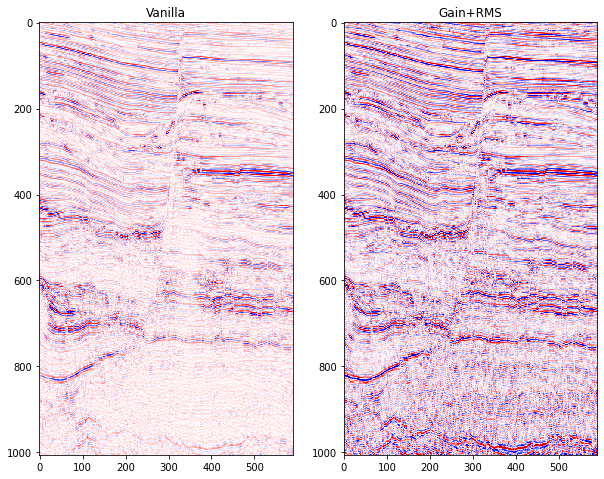Now you can run these to process all the data, but it'll take some time to finish!

In [ ]:
```print('preprocessing the data :')
f = IntProgress(min=0, max=train_data_full.shape)
display(f)
for i in range(0,train_data_full.shape):
#print('reprocess : ',i+1,'of',train_data_full.shape)
train_data_full[:,i,:]=gain(train_data_full[:,i,:],3e-3,0.8)
f.value += 1
```

if you don't want to wait, then you can use this instead.

In [ ]:
```!gdown "https://drive.google.com/uc?id=1JZ5LZz_f2Vfg9BxuGGBY9LliJQAAHi_H"
```
```Downloading...
To: /content/data_train_processed.npz
1.73GB [00:13, 132MB/s]
```

then we rescale the data :

In [ ]:
```train_data_full = (train_data_full - train_data_full.min()) / (train_data_full.max() - train_data_full.min())
```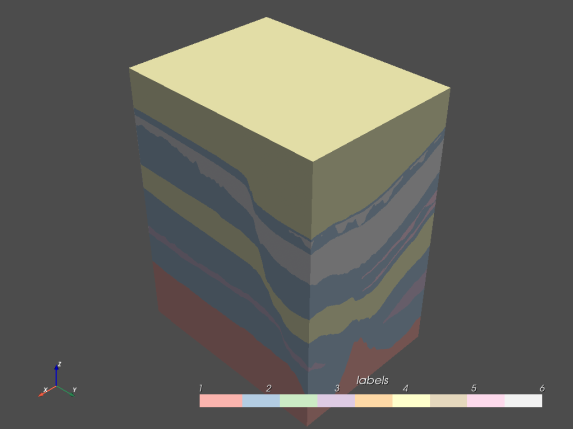And let's see the label distribution :

In [ ]:
```fig = plt.figure(figsize=(10,5))
labels    = ["1", "2", "3", "4", "5", "6"]
colors = ['hotpink', 'lightskyblue', 'mediumpurple','cornsilk', 'pink', 'lightgrey']
N, bins, patches = plt.hist(train_label_full.flatten(),6,density=True, edgecolor='gray', linewidth=1)
for i in range(6):
patches[i].set_facecolor(colors[i])
patches[i].set_label(labels[i])
plt.gca().axes.xaxis.set_ticklabels([])
plt.title('Full Train Data Dist.')
plt.show()
```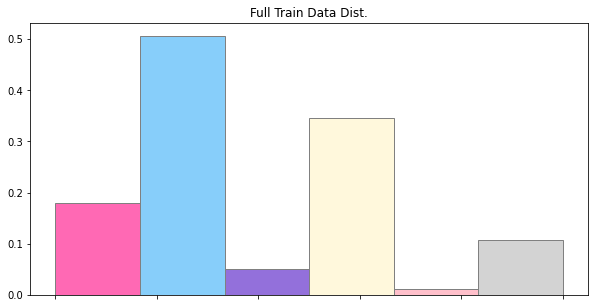Now, about the test cube, it's better to pick the part that also got the label-5.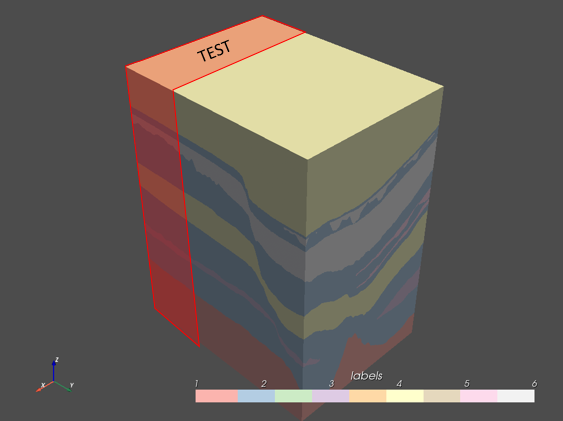Don't do this :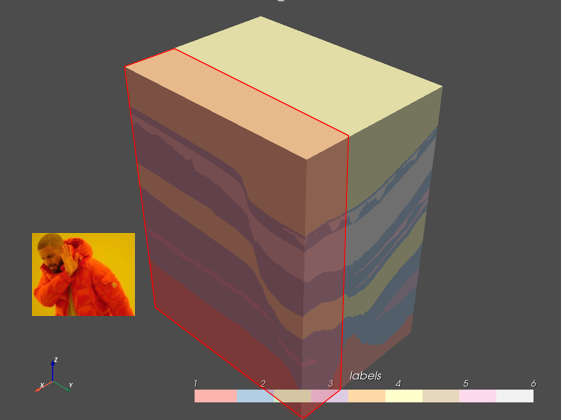As you can see, if we pick this area as a test, then no label-5 that'll be represented.

In [ ]:
```from matplotlib.patches import Rectangle
fig, ax = plt.subplots(figsize=(5,10))
im = ax.imshow(train_label_full[:,-1,:],interpolation='none',cmap='Pastel1')
rect = plt.Rectangle((420,0),300,1500,facecolor='red',alpha=0.5)
plt.show()
```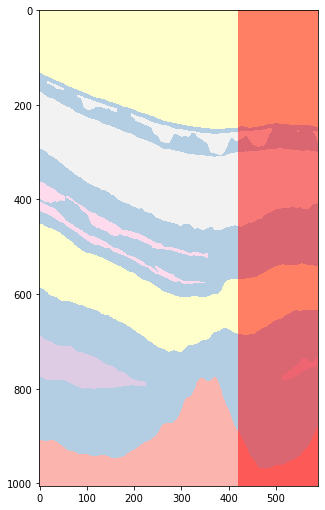Now let's split the train and test data :

In [ ]:
```split_sample = 0.7
test_data = train_data_full[:,:train_data_full.shape-int(train_data_full.shape*split_sample),:]
train_data = train_data_full[:,train_data_full.shape-int(train_data_full.shape*split_sample):,:]
test_label = train_label_full[:,:train_label_full.shape-int(train_label_full.shape*split_sample),:]
train_label = train_label_full[:,train_label_full.shape-int(train_label_full.shape*split_sample):,:]
```
In [ ]:
```print('train cube shape :',train_data.shape)
print('test cube shape :',test_data.shape)
```
```train cube shape : (1006, 547, 590)
test cube shape : (1006, 235, 590)
```
In [ ]:
```fig = plt.figure(figsize=(10,10))
plt.imshow(train_label[:,-1,:],interpolation='none',cmap='Pastel1')
plt.colorbar()
plt.show()
```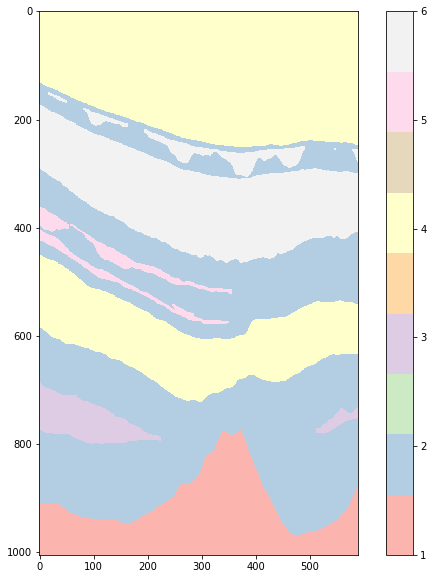and see the label distribution :

In [ ]:
```fig, ax = plt.subplots(1, 2, figsize=(10,5))
labels    = ["1", "2", "3", "4", "5", "6"]
colors = ['hotpink', 'lightskyblue', 'mediumpurple','cornsilk', 'pink', 'lightgrey']
N, bins, patches = ax.hist(train_label.flatten(),[1, 2, 3, 4, 5, 6, 7],density=True, edgecolor='gray', linewidth=1)
for i in range(6):
patches[i].set_facecolor(colors[i])
N2, bins2, patches2 = ax.hist(test_label.flatten(),[1, 2, 3, 4, 5, 6, 7],density=True, edgecolor='gray', linewidth=1)
for i in range(6):
patches2[i].set_facecolor(colors[i])
patches2[i].set_label(labels[i])
ax.get_xaxis().set_visible(False)
ax.get_xaxis().set_visible(False)
ax.set_title("Training Set Dist.")
ax.set_title("Testing Set Dist.")
plt.legend(title="Label")
plt.show()
```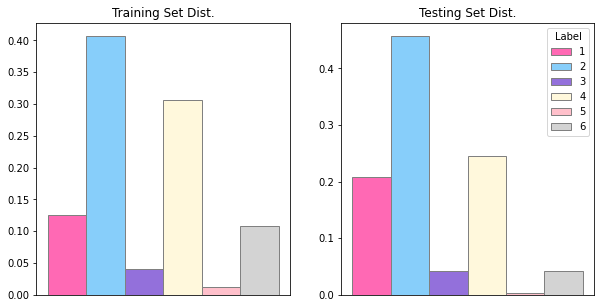Yikes, that's still quite an imbalance that we got here.

In [ ]:
```print('label 5 count of train data : ',np.count_nonzero(train_label.flatten() == 5))
print('label 5 count of test data : ',np.count_nonzero(test_label.flatten() == 5))
```
```label 5 count of train data :  4190167
label 5 count of test data :  428833
```

Still it's not empty if we splice it the other way.

# Setting up the Model, Hyperparameter, Dataloader, etc.¶

Let's set up some stuff for training the models.

First, the metric that we will use from Aicrowd.

In [ ]:
```from sklearn.metrics import multilabel_confusion_matrix
from sklearn.metrics import f1_score,accuracy_score  #for score metric calculation
def _prf_divide(numerator, denominator, ):
"""Performs division and handles divide-by-zero.
On zero-division, sets the corresponding result elements equal to
0 or 1 (according to ``zero_division``).
"""
denominator = denominator.copy()
denominator[mask] = 1  # avoid infs/nans
result = numerator / denominator

return result

def compute_scores(y_true, y_pred, class_weights=[1, 1, 1, 1, 20, 20]):
"""
Computes the weighted & unweighted f1_score and accuracy
Using the standard F1-Score and class-wise accuracy computations were quite
slow as we were doing a lot of redundant work across all score computations,
hence we have implemented this from the base principles.
"""

# Initial Housekeeping Taks1
y_true = np.array(y_true).flatten()
y_pred = np.array(y_pred).flatten()
class_weights = np.array(class_weights)
# print(np.max(y_true))
# print(np.max(y_pred))
# print(np.min(y_true))
# print(np.min(y_pred))
# Computing Multilabel Confusion Matrix
#print("--------- Computing MCM... ")
#begin_time = time.time()
MCM = multilabel_confusion_matrix(y_true, y_pred,labels=[1,2,3,4,5,6])
#print("MCM computation time  : ", time.time() - begin_time)

"""
Gather True Positives, True Negatives, False Positives, False Negatives
"""
tp_sum = MCM[:, 1, 1]
tn_sum = MCM[:, 0, 0]
fn_sum = MCM[:, 1, 0]
fp_sum = MCM[:, 0, 1]

#print("--------- Computing per class instances... ")
per_class_instances = np.bincount(y_true) # Helps keep a track of total number of instances per class
per_class_instances = per_class_instances[1:] # as the class names in the dataset are NOT zero-indexed

assert class_weights.shape == per_class_instances.shape

#print("--------- Computing precision... ")
# precision : tp / (tp + fp)
precision = _prf_divide(
tp_sum,
(tp_sum + fp_sum)
)
#print("--------- Computing recall... ")
# recall : tp / (tp + fn)
recall = _prf_divide(
tp_sum,
(tp_sum + fn_sum)
)

#print("--------- Computing F1 score... ")
# f1 : 2 * (recall * precision) / (recall + precision)
f1_score = _prf_divide(
2 * precision * recall,
precision + recall
)
#print("--------- Computing Accuracy... ")
# accuracy = tp_sum / instances_per_class
# NOTE: we are computing the accuracy independently for all the class specific subgroups
# accuracy = _prf_divide(
#                 tp_sum,
#                 per_class_instances
#             )
# print(class_weights)
# print(f1_score)
f1_score_weighted = np.dot(class_weights, f1_score) / np.sum(class_weights)
f1_score_unweighted = f1_score.mean()

# accuracy_weighted = np.dot(class_weights, accuracy) / np.sum(class_weights)
# accuracy_unweighted = accuracy.mean()

return f1_score_weighted, f1_score_unweighted#, accuracy_weighted, f1_score_unweighted, accuracy_unweighted
```

Then the training parameters:

In [ ]:
```batch_size = 8
num_epochs = 40
num_classes = 6
learning_rate = 0.00085
```

Set up the architecture, pretty simple using `smp`, right?

Also we don't use pretrained weight here. (After some experiment, pretrained weight got worse score).

In [ ]:
```model = smp.PSPNet(
encoder_name="efficientnet-b3",
encoder_weights=None,
in_channels=1,
classes=num_classes,
)
device = torch.device('cuda' if torch.cuda.is_available() else 'cpu')
model.to(device)
print("")
```

Now let's put some random value to test if it works:

In [ ]:
```test = torch.rand(1, 1, 320, 320).cuda()
out = model(test)
out.shape
```
Out[ ]:
`torch.Size([1, 6, 320, 320])`

And set up the optimizer and loss function:

In [ ]:
```criterion = torch.nn.CrossEntropyLoss()
```

Next, let's make the train dataloader:

In [ ]:
```class seisdataset_train(Dataset):
def __init__(self, x_set, y_set):
self.x, self.y = x_set, y_set
self.n_sample = self.x.shape+self.x.shape+self.x.shape
self.aug = A.Compose([
A.Resize(p=1, height=640, width=320, interpolation=1)
])
self.aug2 = A.Compose([
#A.RandomSizedCrop(p=1.0, min_max_height=(1006, 1006), height=1006, width=256, w2h_ratio=1.0, interpolation=0),
#A.GridDistortion(p=0.3, num_steps=6, distort_limit=(-0.2, -0.05), border_mode=1),
A.ElasticTransform(p=0.2,alpha=100, sigma=8, alpha_affine=0, border_mode=1),
A.ShiftScaleRotate(p=0.5, shift_limit=(0.0, 0.0), scale_limit=(0.01, 0.25), rotate_limit=(-15, 15), interpolation=0, border_mode=1),
A.RandomCrop(900, 250, p=0.3),
A.Resize(p=1, height=640, width=320, interpolation=0)

])

def __len__(self):
return self.n_sample

def __getitem__(self, index):
if index < self.x.shape:
idx = index
batch_x = self.x[:,idx,:]
batch_y = self.y[:,idx,:]-1
elif self.x.shape <= index < (self.x.shape+self.x.shape):
idx = index-self.x.shape
batch_x = self.x[:,:,idx]
batch_y = self.y[:,:,idx]-1
elif index >= self.x.shape+self.x.shape:
idx = index-self.x.shape-self.x.shape
batch_x = self.x[:,idx,:]
batch_y = self.y[:,idx,:]-1
```

Wait, what are you doing with albumentation there?

We try to augment some new data.

Well augmentation is.. making new data from available dataset by adding image manipulation algorithm like:

• flip
• rotation
• scale
• elastic transform